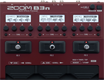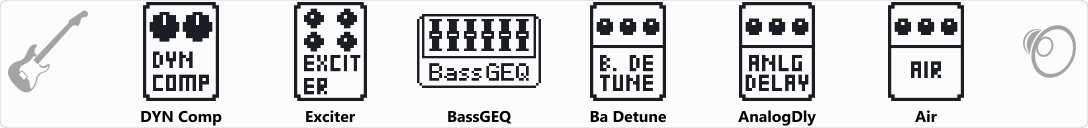# Factory : Tapping

Discussion in 'Zoom B3n' started by Tonelib, May 20, 2018.

1. Factory : TappingDevice: Zoom B3n
Firmware: 2.00

Name on device: Tapping
Optimized for: Phones/Speaker

Effects chain:This setting effectively brings out the sound of tapping.

Effect: "DYN Comp" (Dynamics), active - "yes"
"Sense" = 8
"Attack" = Fast
"Tone" = 65
"Volume" = 50

Effect: "Exciter" (Filter), active - "yes"
"Bass" = 0
"Treble" = 28
"Volume" = 80
"ON/OFF" = LATCH

Effect: "BassGEQ" (Filter), active - "yes"
"50Hz" = -3.0
"120Hz" = -1.5
"400Hz" = -1.0
"500Hz" = 3.0
"800Hz" = 3.0
"4.5kHz" = 4.5
"10kHz" = 4.0
"Volume" = 80
"50Hz" = 0.0
"120Hz" = 0.0
"400Hz" = 0.0
"500Hz" = 0.0
"800Hz" = 0.0
"4.5kHz" = 0.0
"10kHz" = 0.0
"Volume" = 80
"Parameter set" = A

Effect: "Ba Detune" (Modulation), active - "yes"
"Cent" = 8
"PreD" = 0
"Tone" = 6
"Mix" = 60

Effect: "AnalogDly" (Delay), active - "yes"
"Time" = 314
"F.B." = 42
"Mix" = 36
"Tail" = Off

Effect: "Air" (Reverb), active - "yes"
"Size" = 52
"REF" = 5
"Mix" = 53
"Tail" = Off

Patch Volume: 100